# Recent Activity

## Few subsequence sums in Z_n x Z_n ★★

Conjecture   For every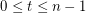, the sequence inconsisting ofcopes ofandcopies of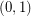has the fewest number of distinct subsequence sums over all zero-free sequences fromof length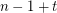.

Keywords: subsequence sum; zero sum

## Olson's Conjecture ★★

Author(s): Olson

Conjecture   Ifis a sequence of elements from a multiplicative group of order, then there exist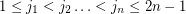so that.

Keywords: zero sum

## Jorgensen's Conjecture ★★★

Author(s): Jorgensen

Conjecture   Every 6-connected graph without a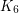minor is apex (planar plus one vertex).

Keywords: connectivity; minor

## Highly connected graphs with no K_n minor ★★★

Author(s): Thomas

Problem   Is it true for all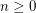, that every sufficiently large-connected graph without a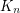minor has a set of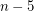vertices whose deletion results in a planar graph?

Keywords: connectivity; minor

## The Alon-Tarsi basis conjecture ★★

Author(s): Alon; Linial; Meshulam

Conjecture   If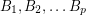are invertiblematrices with entries infor a prime, then there is asubmatrix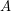of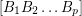so thatis an AT-base.

## The permanent conjecture ★★

Author(s): Kahn

Conjecture   Ifis an invertiblematrix, then there is ansubmatrix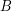ofso thatis nonzero.

Keywords: invertible; matrix; permanent

## The additive basis conjecture ★★★

Author(s): Jaeger; Linial; Payan; Tarsi

Conjecture   For every prime, there is a constant(possibly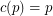) so that the union (as multisets) of anybases of the vector space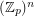contains an additive basis.

## A nowhere-zero point in a linear mapping ★★★

Author(s): Jaeger

Conjecture   Ifis a finite field with at least 4 elements andis an invertiblematrix with entries in, then there are column vectorswhich have no coordinates equal to zero such that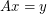.

Keywords: invertible; nowhere-zero flow

## Partitioning edge-connectivity ★★

Author(s): DeVos

Question   Letbe an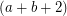-edge-connected graph. Does there exist a partitionof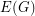so thatis-edge-connected andis-edge-connected?

Keywords: edge-coloring; edge-connectivity

## Acyclic edge-colouring ★★

Author(s): Fiamcik

Conjecture   Every simple graph with maximum degreehas a proper-edge-colouring so that every cycle contains edges of at least three distinct colours.

Keywords: edge-coloring

## Packing T-joins ★★

Author(s): DeVos

Conjecture   There exists a fixed constant(probably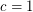suffices) so that every graft with minimum-cut size at leastcontains a-join packing of size at least.

Keywords: packing; T-join

## Decomposing eulerian graphs ★★★

Author(s):

Conjecture   Ifis a 6-edge-connected Eulerian graph andis a 2-transition system for, thenhas a compaible decomposition.

Keywords: cover; cycle; Eulerian

## Faithful cycle covers ★★★

Author(s): Seymour

Conjecture   Ifis a graph,is admissable, and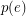is even for every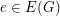, thenhas a faithful cover.

Keywords: cover; cycle

## (m,n)-cycle covers ★★★

Author(s): Celmins; Preissmann

Conjecture   Every bridgeless graph has a (5,2)-cycle-cover.

Keywords: cover; cycle

## The circular embedding conjecture ★★★

Author(s): Haggard

Conjecture   Every 2-connected graph may be embedded in a surface so that the boundary of each face is a cycle.

Keywords: cover; cycle

## Unit vector flows ★★

Author(s): Jain

Conjecture   For every graphwithout a bridge, there is a flow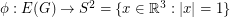.

Conjecture   There exists a mapso that antipodal points of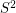receive opposite values, and so that any three points which are equidistant on a great circle have values which sum to zero.

Keywords: nowhere-zero flow

## A homomorphism problem for flows ★★

Author(s): DeVos

Conjecture   Letbe abelian groups and letand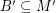satisfy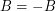and. If there is a homomorphism from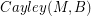to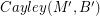, then every graph with a B-flow has a B'-flow.

Keywords: homomorphism; nowhere-zero flow; tension

## The three 4-flows conjecture ★★

Author(s): DeVos

Conjecture   For every graphwith no bridge, there exist three disjoint sets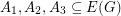withso that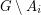has a nowhere-zero 4-flow for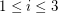.

Keywords: nowhere-zero flow

## Bouchet's 6-flow conjecture ★★★

Author(s): Bouchet

Conjecture   Every bidirected graph with a nowhere-zero-flow for some, has a nowhere-zero-flow.

Keywords: bidirected graph; nowhere-zero flow

## Jaeger's modular orientation conjecture ★★★

Author(s): Jaeger

Conjecture   Every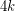-edge-connected graph can be oriented so that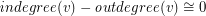(mod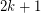) for every vertex.

Keywords: nowhere-zero flow; orientation